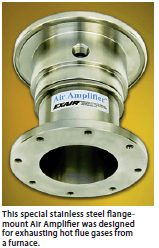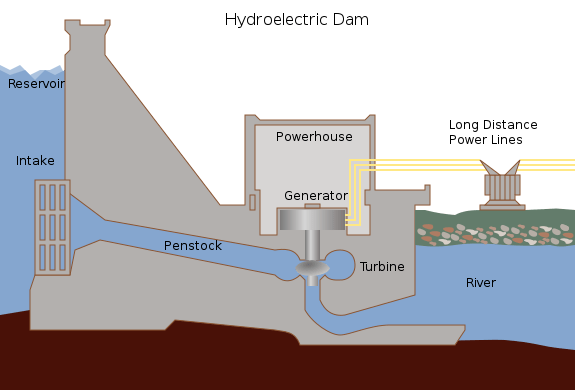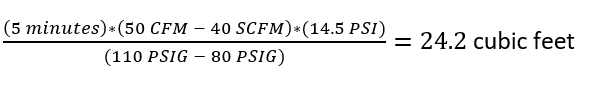## How to Size a Receiver Tank and Improve your Compressed Air System

My colleague, Lee Evans, wrote a blog about calculating the size of primary receiver tanks within a compressed air system.  (You can read it here: Receiver Tank Principle and Calculations).  I would like to expand a bit more about secondary receiver tanks.  They can be strategically placed throughout the plant to improve your compressed air system.  The primary receiver tanks help to protect the supply side when demands are high, and the secondary receiver tanks help systems on the demand side to optimize performance.

I like to compare the pneumatic system to an electrical system.  The receiver tanks are like capacitors.  They store energy produced by an air compressor like a capacitor stores energy from an electrical source.  If you have ever seen an electrical circuit board, you notice many capacitors with different sizes throughout the circuit board (reference photo above).  The reason is to have a ready source of energy to increase efficiency and speed for the ebbs and flows of electrical signals.  The same can be said for the secondary receiver tanks in a pneumatic system.

To tie this to a compressed air system, if you have an area that requires a high volume of compressed air intermittently, a secondary receiver tank would benefit this system.  There are valves, cylinders, actuators, and pneumatic controls which turn on and off.  And in most situations, very quickly.  To maximize speed and efficiency, it is important to have a ready source of air nearby to supply the necessary amount quickly.

For calculating a minimum volume size for your secondary receiver tank, we can use Equation 1 below.  It is the same as sizing a primary receiver tank, but the scalars are slightly different.  The secondary receivers are located to run a certain machine or area.  The supply line to this tank will typically come from a header pipe that supplies the entire facility.  Generally, it is smaller in diameter; so, we have to look at the air supply that it can feed into the tank.  For example, a 1” NPT Schedule 40 Pipe at 100 PSIG can supply a maximum of 150 SCFM of air flow.  This value is used for Cap below.  C is the largest air demand for the machine or targeted area that will be using the tank.  If the C value is less than the Cap value, then a secondary tank is not needed.  If the Cap is below the C value, then we can calculate the smallest volume that would be needed.  The other value is the minimum tank pressure.  In most cases, a regulator is used to set the air pressure for the machine or area.  If the specification is 80 PSIG, then you would use this value as P2.  P1 is the header pressure that will be coming into the secondary tank.  With this collection of information, you can use Equation 1 to calculate the minimum tank volume.  So, any larger volume would fit the requirement as a secondary receiver tank.

Secondary Receiver tank capacity formula (Equation 1)

V = T * (C – Cap) * (Pa) / (P1-P2)

Where:

V – Volume of receiver tank (cubic feet)

T – Time interval (minutes)

C – Air demand for system (cubic feet per minute)

Cap – Supply value of inlet pipe (cubic feet per minute)

Pa – Absolute atmospheric pressure (PSIA)

P2 – Regulated Pressure (PSIG)

If you find that your pneumatic devices are lacking in performance because the air pressure seems to drop during operation, you may need to add a secondary receiver to that system.  For any intermittent design, the tank can store that energy like a capacitor to optimize the performance.  EXAIR stocks 60 Gallon tanks, model 9500-60 to add to those specific locations, If you have any questions about using a receiver tank in your application, primary or secondary, you can contact an EXAIR Application Engineer.  We can restore that efficiency and speed back into your application.

John Ball
Application Engineer
Email: johnball@exair.com

Photo: Circuit Board courtesy from T_Tide under Pixabay License

My colleague, Lee Evans, wrote a blog about calculating the size of receiver tanks within a compressor air system.  (You can read it here: Receiver Tank Principle and Calculations).  But, what if you want to use them in remote areas or in emergency cases?  During these situations, the air compressor is not putting any additional compressed air into the tank.  But, we still have potential energy stored inside the tanks similar to a capacitor that has stored voltage in an electrical system.  In this blog, I will show how you can calculate the size of receiver tanks for applications that are remote or for emergency systems.

From Lee Evans’ blog, Equation 1 can be adjusted to remove the input capacity from an air compressor.  This value is Cap below.  During air compressor shutdowns or after being filled and removed, this value becomes zero.

Receiver tank capacity formula (Equation 1)

V = T * (C – Cap) * (Pa) / (P1-P2)

V – Volume of receiver tank (cubic feet)

T – Time interval (minutes)

C – Air requirement of demand (cubic feet per minute)

Cap – Compressor capacity (cubic feet per minute)

Pa – Absolute atmospheric pressure (PSIA)

P1 – Tank pressure (PSIG)

P2 = minimum tank pressure (PSIG)

Making Cap = 0, the new equation for this type of receiver tank now becomes Equation 2.

Receiver tank capacity formula (Equation 2)

V = T * C * (Pa) / (P1-P2)

With Equation 2, we can calculate the required volume of a receiver tank after it has been pre-charged.  For example, EXAIR created a special Air Amplifier to remove toxic fumes from an oven.  The Air Amplifier was positioned in the exhaust stack and would only operate during power failures.  In this situation, product was being baked in an oven.  The material had toxic chemicals that had to cross-link to harden.  If the power would go out, then the product in the oven would be discarded, but the toxic fumes had to be removed.  What also doesn’t work during power outages is the air compressor.  So, they needed to have a receiver tank with enough volume to store compressed air.  From the volume of the oven, we calculated that they need the special Air Amplifier to operate for 6 minutes.  The compressed air system was operating at 110 PSIG, and the Air Amplifier required an average air flow of 10 cubic feet per minute from the range of 110 PSIG to 0 PSIG.  We are able to calculate the required receiver volume to ensure that the toxic fumes are evacuated from the oven in Equation 2.Receiver tank capacity formula (Equation 2)

V = T * C * Pa / (P1 – P2)

V = 6 minutes * 10 cubic feet per minute * 14.7 PSIA / (110 PSIG – 0 PSIG)

V = 8 cubic feet.

Receiver tanks are more commonly sized in gallons.  In converting 8 cubic feet to gallons, we get a 60-Gallon Receiver Tank.  EXAIR recommended the model 9500-60 to be used near the oven to operate the special Air Amplifier during power outage.

Another way to look at Equation 2 is to create a timing equation.  If the volume of the tank is known, we can calculate how long a system will last.  In this example for scuba diving, we can use this information to configure the amount of time that a tank will last.  The diver has a 0.39 cubic feet tank at a pressure of 3,000 PSIG.  I will use a standard Surface Consumption Rate, SCR, at 0.8 cubic feet per minute.  If we stop the test when the tank reaches a pressure of 1,000 PSIG, we can calculate the time by using Equation 3.

Receiver tank timing formula (Equation 3):

T = V * (P1 – P2) / (C * Pa)

T – Time interval (minutes)

V – Volume of receiver tank (cubic feet)

C – Air demand (cubic feet per minute)

Pa – Absolute atmospheric pressure (PSIA)

P1 – Initial tank pressure (PSIG)

P2 – Ending tank pressure (PSIG)

By placing the values in the Equation 3, we can calculate the time to go from 3,000 PSIG to 1,000 PSIG by breathing normal at the surface.

T = 0.39 cubic feet * (3,000 PSIG – 1,000 PSIG) / (0.8 cubic feet per minute * 14.7 PSIA)

T = 66 minutes.

What happens if the diver goes into deeper water?  The atmospheric pressure, Pa, changes.  If the diver goes to 100 feet below the surface, this is roughly 3 atmospheres or (3 * 14.7) = 44.1 PSIA.  If we use the same conditions above except at 100 feet below, the time will change by a third, or in looking at Equation 3:

T = 0.39 cubic feet * (3,000 PSIG – 1,000 PSIG) / (0.8 cubic feet per minute * 44.1 PSIA)

T = 22 minutes.

If you have any questions about using a receiver tank in your application, you can contact an EXAIR Application Engineer.  We will be happy to solve for the proper volume or time needed for your application.

John Ball
Application Engineer
Email: johnball@exair.com

## Receiver Tank Principle and CalculationsA receiver tank is a form of dry compressed air storage in a compressed air system.  Normally installed after drying and filtration, and before end use devices, receiver tanks help to store compressed air.  The compressed air is created by the supply side, stored by the receiver tank, and released as needed to the demand side of the system.

But how does this work?

The principle behind this concept is rooted in pressure differentials.  Just as we increase pressure when reducing volume of a gas, we can increase volume when reducing pressure.  So, if we have a given volume of compressed air at a certain pressure (P1), we will have a different volume of compressed air when converting this same air to a different pressure (P2).

This is the idea behind a receiver tank.  We store the compressed air at a higher pressure than what is needed by the system, creating a favorable pressure differential to release compressed air when it is needed.  And, in order to properly use a receiver tank, we must be able to properly calculate the required size/volume of the tank.  To do so, we must familiarize ourselves with the receiver tank capacity formula.

V = ( T(C-Cap)(Pa)/(P1-P2) )

Where,

V = Volume of receiver tank in cubic feet

T = Time interval in minutes during which compressed air demand will occur

C = Air requirement of demand in cubic feet per minute

Cap = Compressor capacity in cubic feet per minute

Pa = Absolute atmospheric pressure, given in PSIA

P1 = Initial tank pressure (Compressor discharge pressure)

P2 = minimum tank pressure (Pressure required at output of tank to operate compressed air devices)

An example:

Let’s consider an application with an intermittent demand spike of 50 SCFM of compressed air at 80 PSIG.  The system is operating from a 10HP compressor which produces 40 SCFM at 110 PSIG, and the compressed air devices need to operate for (5) minutes at this volume.

We can use a receiver tank and the pressure differential between the output of the compressor and the demand of the system to create a reservoir of compressed air.  This stored air will release into the system to maintain pressure while demand is high and rebuild when the excess demand is gone.

In this application, the values are as follows:

V = ?

T = 5 minutes

C = 50 CFM

Cap = 40 SCFM

Pa = 14.5 PSI

P1 = 110 PSIG

P2 = 80 PSIG

Running these numbers out we end up with:This means we will need a receiver tank with a volume of 24.2 ft.³ (24.2 cubic feet equates to approximately 180 gallons – most receiver tanks have capacities rated in gallons) to store the required volume of compressed air needed in this system.  Doing so will result in a constant supply of 80 PSIG, even at a demand volume which exceeds the ability of the compressor.  By installing a properly sized receiver tank with proper pressure differential, the reliability of the system can be improved.

This improvement in system reliability translates to a more repeatable result from the compressed air driven devices connected to the system.  If you have questions about improving the reliability of your compressed air system, exactly how it can be improved, or what an engineered solution could provide, contact an EXAIR Application Engineer.  We’re here to help.

Lee Evans
Application Engineer
LeeEvans@EXAIR.com
@EXAIR_LE

Tennessee Valley Authority; SVG version by Tomia, CC BY-SA 3.0 <http://creativecommons.org/licenses/by-sa/3.0/>, via Wikimedia Commons